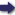(London :  Kegan Paul, Trench, Trübner & Co.,  1910.)

 Tools

## Search this bookPrev Page 366 Next``` 366 ALBERUNTS INDIA. no month, but only complete years; therefore we have nothing to add to this number. It represents the par¬ tial solar months. We multiply it by 53ii and divide the product by 172,800; the quotient 727,661,6331!-^ represents the adhimasa months. Omitting the frac¬ tions, we add 727,661,633 to the partial solar months 23,675,377,584, and get 24,403,039,217 as the partial lunar months. By multiplying this number by 30 we get days, viz., 732,091,176,510. As there are no days in the normal date, we have no days to add to this number. Multiplying it by 55,739 and dividing the pro¬ duct by 3,562,220, we get the partial unaratra days, viz., 11,455,224,575i|-ff|f. This sum of days without the fraction is subtracted from the partial lunar days, and the remainder, 720,635,951,935, represents the number of the civil days of our gauge-date. Dividing it by 7, we get as remainder 4, which means that the last of these days is a Wednesday. Therefore the Indian year commences with a Thursday, The difference between 720,635,951,935 and the beginning of the kaliyuga 720,634,442,715 is, as it ought to be, 1,509,220 days (Schrctm). In the beginning of chap. Iii., in the Arabic text, r H. 8, it seems necessary to write j»i-^ and j^i-^^^ instead of T^ and ^[.'"j]. i •• P, 29, 1. 10. Thursdety.—The Arabic manuscript has Tuesdary. P. 30,1. 10-17.—This ought to run as follows :—We have found above 727,661,633||-||- for the adhimasa months ; the wholes represent the number of the adhimasas which have elapsed, viz., 727,661,633, whilst the fraction is the time which has already elapsed of the current adhimasa month. By multiplying this fraction by 30 we get it expressed in days, viz., 'Y^if days, or 28 days 51 minutes 30 seconds, so that the current adhimasa month wants only I day 8 minutes 30 seconds more to become a complete month (Schrctm). P, 31, 1, 19.—^The number 1,203,783,270 is found by adding the 30 x 1,196,525 or 35,895,750 adhimasa days to the 1,167,887,520 solar days (Schram). ```Prev Page 366 Next